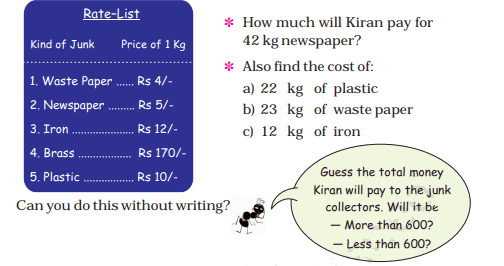# CBSE Class 4 Maths Solutions

The CBSE is an educational institute under the government of India. Most of the private schools and government schools are affiliates to CBSE board. These schools follow the NCERT syllabus and books that are prescribed by the board. The CBSE Class 4 Maths Syllabus is prepared in a strategic way to keep the students engaged with learning while introducing them to a wide variety of topics. Several important topics are introduced in an interesting and easy to understand way. The books include several questions that help the students to get thorough with the concepts taught in the class.

## CBSE Class 4 Maths Solutions

Students must solve the exercise questions that are provided at the end of each chapter. Here, we have provided CBSE class 4 Maths solutions. Check these solutions and clear any confusion that might arise while solving the maths problems. Through this, students will also get to know how to write the step by step solution to fetch more marks in the exam.

Question: [Reference: Chapter 12- How Heavy? How Light?]1. To find the total weight that they had loaded on the cart, it is required to find the total weight of each thing loaded on the cart and add them.
Thing Loaded No. of Items (given in the question) Total Weight
A sack of wheat 5 5 × 100 kg = 500 kg
A sack of rice 3 3 × 35 kg = 105 kg
A water tank 1 = 50 kg
An almirah 1 = 70 kg
A table 3 3 × 10 kg = 30 kg
A chair 4 4 × 5 kg = 20 kg
A mattress 2 2 × 20 kg = 40 kg
Bamboo ladder 1 = 10 kg
Pots and pans 1 = 10 kg
Total weight 21 835 kg

Thus, they had loaded a total of 853 Kg weight on the cart.

1. The will move when the weight equals 700 kg. So, the weight that needs to be removed is (835 – 700) = 135 kg.

By removing 3 sacks of rice, a total of 105 kg is reduced. Also, to remove another 30 kg, 3 tables can be removed which weighs 10 kg each. So, they have to remove 3 sacks of rice and 3 tables to make the total weight of the cart as 700 kg.

Question: [Reference: Chapter 6- The Junk Seller]• As the cost of 1 kg newspaper is Rs. 5, cost of 31 kg newspaper will be = 5 × 31 = 155.

So, Kiran will have to pay Rs. 155 for 31 kg newspaper.

• Similarly, for 42 kg newspaper, she will have to pay 15 × 42 = 210.

Thus, Rs. 210 has to be paid for 42 kg newspaper.

• 1 kg of plastic costs = Rs. 10

So, 22 kg of plastic will cost = Rs. 10 × 22 = Rs. 220.

• 1 kg of waste paper costs Rs. 4

Therefore, 23 kg of waste paper will cost = Rs. 4 × 23 = Rs. 92.

• III. Cost of 1 kg iron = Rs. 12

Hence, 12 kg of iron will cost = Rs. 12 × 12 = Rs. 144.

Keep visiting BYJU’S to get more such solutions, worksheets and sample papers. BYJU’S also provide several question papers and latest notifications to keep the students updated. Students can also visit CBSE class 4 maths worksheets to practice few more question variations and get completely acquainted with all the important maths topics.